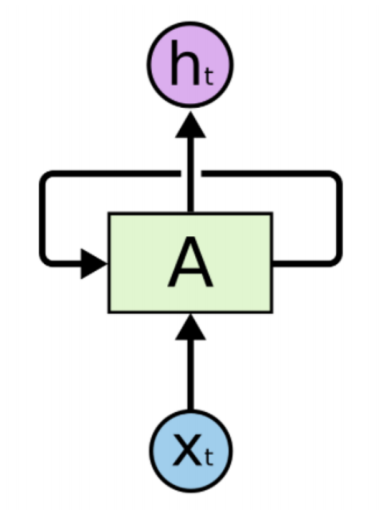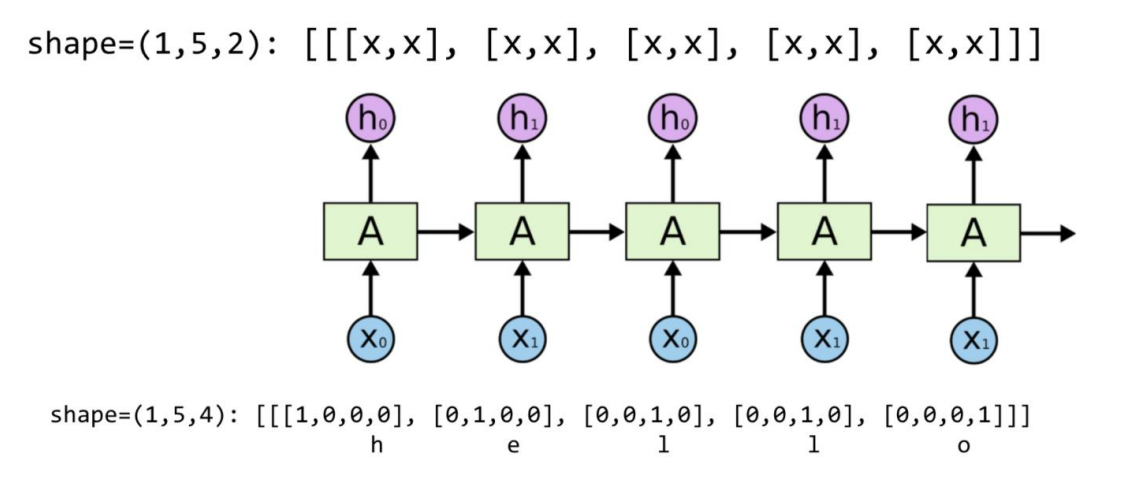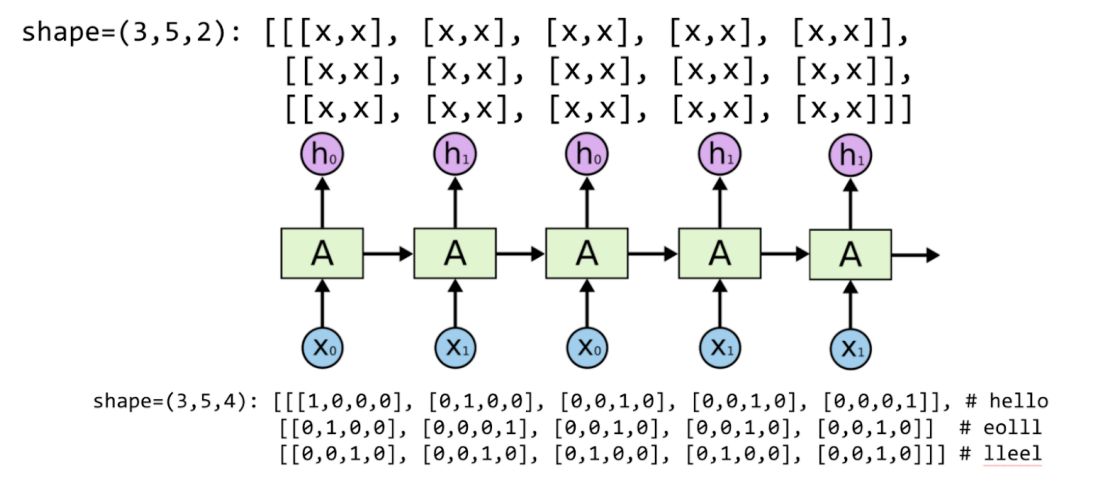import tensorflow as tf
import numpy as np
import matplotlib.pyplot as plt
import pandas as pd

print('Tensorflow: {}'.format(tf.__version__))

plt.rcParams['figure.figsize'] = (16, 10)
plt.rc('font', size=15)

Tensorflow: 2.3.1


## RNN in Tensorflow

Recurrent Neural Network (RNN for short) is the neural network that has backward stream into input node. Simple notation is expressed like this,And it is implemented in Tensorflow (of course, it can be easily used with tensorflow keras). There are two implementation approaches,

• Using basic cell (SimpleRNNCell) and merge it with multiple elements to build complex model like Long Short Term Mermory (LSTM) or Gated Recurrent Unit (GRU)
cell = tf.keras.layers.SimpleRNNCell(units=hidden_size)
rnn = tf.keras.layers.RNN(cell, return_sequences=True, return_state=True)
outputs, states = rnn(X_data)

• Using RNN Layer (SimpleRNN)
rnn = tf.keras.layers.SimpleRNN(units=hidden_size, return_sequences=True,
return_state=True)
outputs, states = rnn(X_data)


## Simple RNN Cell

Let's look at more closely with example. Suppose that we want to train RNN that can generate the word "hello". Of course, the model cannot interpret word itself, so it is required to convert it with one-hot encoding. Currently, "Hello" is the word with 4 characters, "h", "e", "l", "o". So we can define each character with one-hot expression.

h = [1, 0, 0, 0]
e = [0, 1, 0, 0]
l = [0, 0, 1, 0]
o = [0, 0, 0, 1]


In tensorflow, to insert this data, we need to reshape like this order:

$$(\text{batch_size}, \text{sequence_length}, \text{sequence_width})$$

If we want to insert one-hot encoded h in RNN as an input, we can make it with numpy array,

X_data = np.array([[h]], dtype=np.float32)
X_data.shape

(1, 1, 4)

Then, we can make RNN cell with hidden_size of 2.

hidden_size = 2
cell = tf.keras.layers.SimpleRNNCell(units=hidden_size)
rnn = tf.keras.layers.RNN(cell, return_sequences=True, return_state=True)
outputs, states = rnn(X_data)

print("X_data: {}, shape: {}".format(X_data, X_data.shape))
print("output: {}, shape: {}".format(outputs, outputs.shape))
print("states: {}, shape: {}".format(states, states.shape))

X_data: [[[1. 0. 0. 0.]]], shape: (1, 1, 4)
output: [[[-0.6887451  0.2290415]]], shape: (1, 1, 2)
states: [[-0.6887451  0.2290415]], shape: (1, 2)


Mentioned before, we can also build it with layer API.

rnn_2 = tf.keras.layers.SimpleRNN(units=hidden_size, return_sequences=True, return_state=True)
outputs, states = rnn_2(X_data)

print("X_data: {}, shape: {}".format(X_data, X_data.shape))
print("output: {}, shape: {}".format(outputs, outputs.shape))
print("states: {}, shape: {}".format(states, states.shape))

X_data: [[[1. 0. 0. 0.]]], shape: (1, 1, 4)
output: [[[-0.07527272 -0.67188245]]], shape: (1, 1, 2)
states: [[-0.07527272 -0.67188245]], shape: (1, 2)


## Unfolding multiple sequences

But in above example, the output is meaningless. RNN is neural network for sequence data, but we just insert 1-sequence data. In order to understand the RNN operation, we need to insert multiple-length sequences.In this case, we will insert 5-length sequences. So input data shape will be like this,

$$(1, 5, 4)$$

Currently, we just use 1 batch, and 5-length sequence, and our data have 4 width after one-hot encoding. If the hidden size is 2, then the output shape is aligned to hidden_size.

Anyway, Our input data will be like this,

X_data = np.array([[h, e, l, l, o]], dtype=np.float32)
X_data.shape

(1, 5, 4)

Same as before, we can build RNN cell.

cell = tf.keras.layers.SimpleRNNCell(units=hidden_size)
rnn = tf.keras.layers.RNN(cell, return_sequences=True, return_state=True)
outputs, states = rnn(X_data)

print("X_data: {}, shape: {}\n".format(X_data, X_data.shape))
print("output: {}, shape: {}\n".format(outputs, outputs.shape))
print("states: {}, shape: {}".format(states, states.shape))

X_data: [[[1. 0. 0. 0.]
[0. 1. 0. 0.]
[0. 0. 1. 0.]
[0. 0. 1. 0.]
[0. 0. 0. 1.]]], shape: (1, 5, 4)

output: [[[-0.12052011  0.5526149 ]
[ 0.4244858   0.65038365]
[-0.8238987   0.44178498]
[ 0.00774928  0.73565745]
[ 0.3128305   0.8846916 ]]], shape: (1, 5, 2)

states: [[0.3128305 0.8846916]], shape: (1, 2)


Output contains each hidden states while input data is processed in RNN cell. So the the last element in output is the same as states, since it is the latest states after process.

## Batching Input

Of course, we can extend the dataset with batch size.Suppose that we have 3 batch data as inputs, and our input data is hello, eolll, and lleel. Don't worry about complex shape dataset. All the process is the same as before. All you need to do is to make the input dataset with appropriate shape. So in this case, we need to make (3, 5, 4)-shaped input.

X_data = np.array([[h, e, l, l, o],
[e, o, l, l, l],
[l, l, e, e, l]], dtype=np.float32)
X_data.shape

(3, 5, 4)
cell = tf.keras.layers.SimpleRNNCell(units=hidden_size)
rnn = tf.keras.layers.RNN(cell, return_sequences=True, return_state=True)
outputs, states = rnn(X_data)

print("X_data: {}, shape: {}\n".format(X_data, X_data.shape))
print("output: {}, shape: {}\n".format(outputs, outputs.shape))
print("states: {}, shape: {}".format(states, states.shape))

X_data: [[[1. 0. 0. 0.]
[0. 1. 0. 0.]
[0. 0. 1. 0.]
[0. 0. 1. 0.]
[0. 0. 0. 1.]]

[[0. 1. 0. 0.]
[0. 0. 0. 1.]
[0. 0. 1. 0.]
[0. 0. 1. 0.]
[0. 0. 1. 0.]]

[[0. 0. 1. 0.]
[0. 0. 1. 0.]
[0. 1. 0. 0.]
[0. 1. 0. 0.]
[0. 0. 1. 0.]]], shape: (3, 5, 4)

output: [[[-0.0886425  -0.35497615]
[-0.37740785  0.4966906 ]
[-0.17157763  0.7537353 ]
[-0.36729524  0.62280154]
[-0.49442795  0.61391914]]

[[-0.6217956   0.37030688]
[-0.330911    0.767418  ]
[-0.40512574  0.708009  ]
[-0.36787215  0.7476258 ]
[-0.39487016  0.72745013]]

[[ 0.36914107  0.60953295]
[-0.137596    0.22348596]
[-0.7504324   0.44638833]
[-0.86478275  0.77702737]
[-0.49543512  0.88641524]]], shape: (3, 5, 2)

states: [[-0.49442795  0.61391914]
[-0.39487016  0.72745013]
[-0.49543512  0.88641524]], shape: (3, 2)


Mentioned before, output contains each hidden states while processing the input data. Each hidden state affects the next hidden state and so on. Then the last hidden state will be states that outputs from RNN cell.

## Summary

Through this post, we covered simple usage of RNN cell and layers in Tensorflow. We just define the dataset with appropriate format, then the RNN layer can generates hidden states for the output that affects to next state.